# HSSlive: Plus One & Plus Two Notes & Solutions for Kerala State Board

## BSEB Class 9 Maths Chapter 6 Lines and Angles Ex 6.2 Textbook Solutions PDF: Download Bihar Board STD 9th Maths Chapter 6 Lines and Angles Ex 6.2 Book AnswersBSEB Class 9 Maths Chapter 6 Lines and Angles Ex 6.2 Textbook Solutions PDF: Download Bihar Board STD 9th Maths Chapter 6 Lines and Angles Ex 6.2 Book Answers

BSEB Class 9th Maths Chapter 6 Lines and Angles Ex 6.2 Textbooks Solutions and answers for students are now available in pdf format. Bihar Board Class 9th Maths Chapter 6 Lines and Angles Ex 6.2 Book answers and solutions are one of the most important study materials for any student. The Bihar Board Class 9th Maths Chapter 6 Lines and Angles Ex 6.2 books are published by the Bihar Board Publishers. These Bihar Board Class 9th Maths Chapter 6 Lines and Angles Ex 6.2 textbooks are prepared by a group of expert faculty members. Students can download these BSEB STD 9th Maths Chapter 6 Lines and Angles Ex 6.2 book solutions pdf online from this page.

## Bihar Board Class 9th Maths Chapter 6 Lines and Angles Ex 6.2 Books Solutions

 Board BSEB Materials Textbook Solutions/Guide Format DOC/PDF Class 9th Subject Maths Chapter 6 Lines and Angles Ex 6.2 Chapters All Provider Hsslive

## How to download Bihar Board Class 9th Maths Chapter 6 Lines and Angles Ex 6.2 Textbook Solutions Answers PDF Online?

2. Click on the Bihar Board Class 9th Maths Chapter 6 Lines and Angles Ex 6.2 Answers.
3. Look for your Bihar Board STD 9th Maths Chapter 6 Lines and Angles Ex 6.2 Textbooks PDF.
4. Now download or read the Bihar Board Class 9th Maths Chapter 6 Lines and Angles Ex 6.2 Textbook Solutions for PDF Free.

## BSEB Class 9th Maths Chapter 6 Lines and Angles Ex 6.2 Textbooks Solutions with Answer PDF Download

Find below the list of all BSEB Class 9th Maths Chapter 6 Lines and Angles Ex 6.2 Textbook Solutions for PDF’s for you to download and prepare for the upcoming exams:

## BSEB Bihar Board Class 9th Maths Solutions Chapter 6 Lines and Angles Ex 6.2

Question 1.
In figure, find the values of x and y and then show that AB || CD.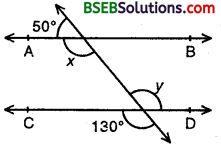Solution:
Since AB || CD and transversal PQ intersects them at R and S respectively.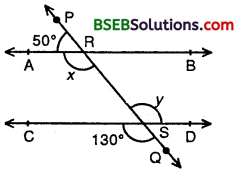∴ ∠ARS = ∠RSD [Alternate angles]
⇒ x = y
But ∠RSD = ∠CSQ
[ Vertically opp. angles]
⇒ y = 130° [∵∠CSQ = 130°]
Hence, x = y = 130°.

Question 2.
In figure, if AB || CD, CD || EF and y : z = 3 : 7, find x.Solution:
Since CD || EF and transversal PQ intersects them at S and T respectively.
∠CST = ∠STF [Alternate Angles]
⇒ 180° – y = z
[∵ ∠y + ∠CST = 180° being linear pair]
⇒ y + z = 180°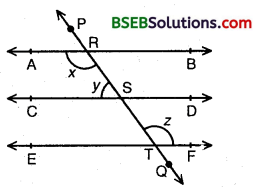Given y : z = 3 : 7.
So, the sum of ratios = 3 + 7 = 10
∴ y = 310 x 180°
= 3 x 18° = 54°
and, z = 710 x 180°
= 7 x 18° = 126°
Since AB || CD and transversal PQ intersects them at R and S respectively.
∴ ∠ARS + ∠RSC = 180° [Consecutive interior angles are supplementary]
⇒ x + y = 180°.
⇒ x = 180° – y
= 180° – 54° = 126° [∵ y = 54°]
Hence x = 126°.

Question 3.
In figure, if AB || CD, EF ⊥ CD and ∠GED = 126°, find ∠AGE, ∠GEF and ∠FGE.Solution:
Since AB || CD and transversal GE cuts them at G and E respectively.
∠AGE = ∠GED [Alternate angles]
∠AGE = 126° [∵ ∠GED = 1260 (given)]
∠GEF = ∠GED – ∠FED = 126° – 90° = 36°
∠FGE = ∠GEC [Alternate angles]
∠FGE = 90° – ∠GEF
= 90° – 36° = 54°
Hence, ∠AGE = 126°, ∠GEF = 36° and ∠FGE = 54°.

Question 4.
In figure, if PQ || ST, ∠PQR = 110° and ∠RST =130°, find ∠QRS.Solution:
Produce PQ to intersect SR in a point M.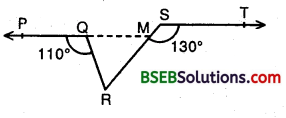Now, PM || ST and transversal SM intersects them at M and R respectively.
∴ ∠SMQ = ∠TSM [Alternate angles]
⇒ ∠SMQ = 130°
⇒ ∠QMR = 180° – 130° = 50° [∵ ∠SMQ + ∠QMR = 180° linear pairs]
Since, ray RQ stands at Q on PM.
∴ ∠PQR + ∠RQM = 180°
⇒ 110° + ∠RQM = 180°
⇒ ∠RQM = 70°
∴ ∠QRS = 180° – (70° + 50°) = 60°
[∵ Sum of the angles of a triangle is 180°]

Question 5.
In figure, if AB || CD, ∠APQ = 50° and ∠PRD = 127°, find x and y.Solution:
∵ AB || CD and transversal PQ intersects them at P and Q respectively.
∴ ∠PQR = ∠APQ [Alternate angles]
⇒ x = 50° [∵ ∠APQ = 50° (given)]
∵ AB || CD and transversal PR intersects them at P and R respectively.
∴ ∠APR = ∠PRD [Alternate angles]
⇒ ∠APQ + ∠QPR = 127° [∵ ∠PRD = 127°]
⇒ 50° + y = 127° [∵∠APQ = 50°]
⇒ y = 127° – 50° = 77°
Hence, x = 50° and y = 11°.

Question 6.
In figure, PQ and RS are two mirrors placed parallel to each other. An incident ray AB strikes the mirror PQ at B, the reflected ray moves along the path BC and strikes the mirror RS at C and again reflects back along CD. Prove that AB || CD.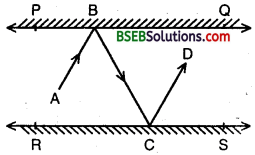Solution:
Two-plane mirrors PQ and RS are placed parallel to each other i.e., PQ || RS. An incident ray AB after reflections takes the path BC and CD.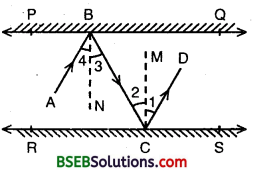BN and CM are the normals to the plane mirrors PQ and RS respective.
Since BN ⊥ PQ, CM ⊥ RS and PQ || RS
∴ BN ⊥ RS ⇒ BN || CM
Thus BN and CM are two parallel lines and a transversal BC cuts them at B and C respectively.
∴ ∠2 = ∠3 [Alternate interior angles]
But, ∠1 = ∠2 and ∠3 = ∠4 [By laws of reflection]
∠1 + ∠2 = ∠2 + ∠2 and ∠3 + ∠4= ∠3 + ∠3
⇒ ∠1 + ∠2 = 2(∠2) and ∠3 + ∠4 = 2(∠3)
⇒ ∠1 + ∠2 = ∠3 + ∠4 [∵ ∠2 = ∠3 ⇒ 2(∠2) = 2(∠3)]
⇒ ∠ABC = ∠BCD
Thus, lines AB and CD are intersected by transversal BC such that
∠ABC = ∠BCD.
i.e., alternate interior angles are equal.
Therefore, AB || CD.

## Bihar Board Class 9th Maths Chapter 6 Lines and Angles Ex 6.2 Textbooks for Exam Preparations

Bihar Board Class 9th Maths Chapter 6 Lines and Angles Ex 6.2 Textbook Solutions can be of great help in your Bihar Board Class 9th Maths Chapter 6 Lines and Angles Ex 6.2 exam preparation. The BSEB STD 9th Maths Chapter 6 Lines and Angles Ex 6.2 Textbooks study material, used with the English medium textbooks, can help you complete the entire Class 9th Maths Chapter 6 Lines and Angles Ex 6.2 Books State Board syllabus with maximum efficiency.

## FAQs Regarding Bihar Board Class 9th Maths Chapter 6 Lines and Angles Ex 6.2 Textbook Solutions

#### How to get BSEB Class 9th Maths Chapter 6 Lines and Angles Ex 6.2 Textbook Answers??

Students can download the Bihar Board Class 9 Maths Chapter 6 Lines and Angles Ex 6.2 Answers PDF from the links provided above.

#### Can we get a Bihar Board Book PDF for all Classes?

Yes you can get Bihar Board Text Book PDF for all classes using the links provided in the above article.

## Important Terms

Bihar Board Class 9th Maths Chapter 6 Lines and Angles Ex 6.2, BSEB Class 9th Maths Chapter 6 Lines and Angles Ex 6.2 Textbooks, Bihar Board Class 9th Maths Chapter 6 Lines and Angles Ex 6.2, Bihar Board Class 9th Maths Chapter 6 Lines and Angles Ex 6.2 Textbook solutions, BSEB Class 9th Maths Chapter 6 Lines and Angles Ex 6.2 Textbooks Solutions, Bihar Board STD 9th Maths Chapter 6 Lines and Angles Ex 6.2, BSEB STD 9th Maths Chapter 6 Lines and Angles Ex 6.2 Textbooks, Bihar Board STD 9th Maths Chapter 6 Lines and Angles Ex 6.2, Bihar Board STD 9th Maths Chapter 6 Lines and Angles Ex 6.2 Textbook solutions, BSEB STD 9th Maths Chapter 6 Lines and Angles Ex 6.2 Textbooks Solutions,
Share: